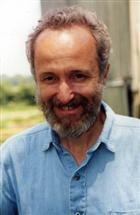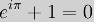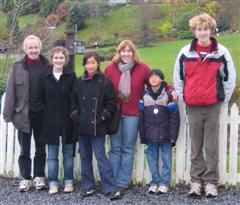# Andrew Rich

## | Mathematics### Andrew F. Rich

Associate Professor of Mathematics

Department of Mathematics and Computer Science

Manchester College

Ph: (260)982-5313

Fax: (260)982-5043

africh@manchester.edu

Courses taught at Manchester

Basic Mathematics (MATH 100)

College Algebra (MATH 112)

Precalculus (MATH 121)

Calculus I (MATH 121)

Discrete Mathematics (MATH 130)

Ordinary Differential Equations (MATH 245)

Linear Algebra I (MATH 251)

History of Mathematics (MATH T31)

Geometry (MATH 306)

Algebraic Structures (MATH 333)

Real Analysis (MATH 421)

Math Sites

MacTutor Math Biographies and History

Math World (Eric Weisstein)

Polking Diff Eq Software

Function Grapher (Walter Zorn)

Links

Manchester College home page

Mathematics & Computer Science Department

Science Seminar

Curriculum VitaeThe Rich family in Scotland, November 2006.